﻿ Attribute Properties > The Record dialog box > The Data field dialog box

# The Data field panel

Navigation:  Attribute Properties > The Record dialog box >

# The Data field panel

The Data field panel contains the following controls:

The Data field panel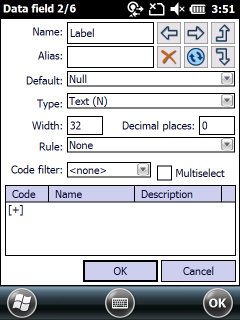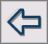- Shows the previous data field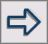- Shows the next data field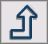- Moves the data field upwards in the Record panel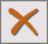- Deletes the current data field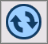- Refreshes the data field values when using a geometry type data field default value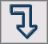- Moves the data field downwards in the Record panel

Name: Shows the editable name of the data field.

Alias: Shows the editable alias name of the data field. Default alias name is equal with the data field name. The alias data field name will be displayed on the Form view.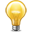The data field alias name can be stored in TopoLynx TAB file format and can be stored in TopoLynx topoXplore Map (.EXP) file in case of using other file formats.

Default: Shows the data field default value that will be used in case of record creation or creating/changing geometry

Null: Field value will be zero/empty

Copy last value: Field value will be the copy of the previous record value

Copy nearest: Field value will be the copy of the nearest feature's record value

Increment last value: Field value will be the increased value of the previous record

Creation time: Field value will be equal to the creation time (the stored value depends on the operation system's time settings)

GPS time: Field value will be the GPS Universal time

Area of Geometry (m2): Field value will be the area of the geometry in square meter on geometry creation/modification

Area of Geometry (ha): Field value will be the area of the geometry in hectare on geometry creation/modification

Area of Geometry (ac): Field value will be the area of the geometry in acre on geometry creation/modification

Area (custom): Field value will be the area of the geometry in current area unit on geometry creation/modification

Perimeter of Geometry (m): Field value will be the perimeter of the geometry in meter on geometry creation/modification

Perimeter of Geometry (km): Field value will be the perimeter of the geometry in kilometer on geometry creation/modification

Perimeter (custom): Field value will be the perimeter of the geometry in current length unit on geometry creation/modification

Length of Geometry (m): Field value will be the length of the geometry in meter on geometry creation/modification

Length of Geometry (km): Field value will be the length of the geometry in kilometer on geometry creation/modification

Length (custom): Field value will be the length of the geometry in current length unit on geometry creation/modification

Distance to last object (custom): Field value will be
- the distance between two point features
- the distance between two line features (distance calculated between the center positions of the middle sections in the line feature)
- the distance of the center's positions between two polygon features in current length unit on geometry creation/modification

Azimuth Angle: Field value will be the angle of the geometry on geometry creation/modification

GPS heading: Field value will be the angle of the current GPS direction

Speed (m/s): Field value will be the current calculated GPS speed in m/s

Speed (custom): Field value will be the current calculated GPS speed in current speed unit

Easting coordinate: Field value will be the X coordinate of the geometry on geometry creation/modification

Northing coordinate: Field value will be the Y coordinate of the geometry on geometry creation/modification

Latitude: Field value will be the Latitude coordinate of the geometry on geometry creation/modification

Longitude: Field value will be the Longitude coordinate of the geometry on geometry creation/modification

Altitude: Field value will be the height of the geometry = Mean Sea Level (MSL) if a geoid separation value is specified

Height above ellipsoid: (HAE) Field value will be the height above the ellipsoid

Satellites in use: Field value will be the number of used satellites

Satellites in view: Field value will be the number of viewable satellites

PDOP value: Field value will be the PDOP value

Horizontal error: Field value will be the horizontal RMS error (horizontal positioning error)

Vertical error: Field value will be the vertical RMS error (horizontal positioning error)

DGPS fix rate: Field value will be the ratio of the DGPS fix positions (in percent) proportion to uncorrected positions

PPS fix rate: Field value will be the ratio of the PPS fix positions (in percent) proportion to uncorrected positions

FloatRTK rate: Field value will be the ratio of the FloatRTK positions (in percent) proportion to uncorrected positions

FixRTK rate: Field value will be the ratio of the FixRTK positions (in percent) proportion to uncorrected positions

Differential Correction Age: Field value will be the differential correction age

Correction type: Field value will be the ratio of differentially corrected positions (in percent) proportion to corrected positions (e.g. 70% Autonomous, 30% DGPS)

Measure reliability: Reliability value calculated by the PDOP values

Measure limit: Reliability limit calculated by the measured area

Measure identifier: Field value will be a unique identifier of the primary measured feature during the GPS data capture. Required when you collect attributes for vertices in a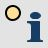point info layer

Unique identifier (GUID): Field value will be a GUID unique identifier of the primary measured feature during the GPS data capture. Required to use it in multi-user environment instead of the Measure identifier default field value. Required when you collect attributes for vertices in apoint info layer

Username: Field value will be the logged in user to the LOGIN module. (The LOGIN module is an optional module to TopoLynx topoXplore, can be purchased additionally.)The data field default value can be stored in TopoLynx TAB file format and can be stored in the TopoLynx topoXplore Map (.EXP) file in case of using other file formats.

Type: Shows the data type of the field.

Logical: 1 byte length logical (true/false) value

Byte: 1 byte length integer value. Value range: 0..255

Short integer: 2 byte length integer value. Value range: -32768..32767

Long integer: 2 byte length integer value. Value range: -2147483648..2147483647

Float: 4 byte length real value provides up to 7 decimal digits

Double: 8 byte length real value provides up to 15 decimal digits

Date: 4 byte length date with minute precision

Time: 8 byte length date with 1/10000 second precision

Text: Character field without size limitThe maximum character size to Text field type is 254 character in DBF file format (to ESRI Shape files).

Document: This type stores a path or URL of an object (image, sound, document) by using the Document panel

Width: Shows the number of the characters to the field name in case of fixed record size formats (DBF, TAB)The maximum field name width is 11 character in DBF file format (to ESRI Shape files)

Decimal places: Number of decimals to display real values

Rule: Verification procedure after each modification of the field value

None: No verification

Read only: The cell value cannot be modified. Recommended for calculated fields

Not null: The cell value cannot be null

Unique value: The cell value cannot be repeated, must be unique in the table

Range: The cell value must be in a given range (between minimum and maximum)

Valid value: The cell value must be an existing value of the data field

Code filter: Filters the selectable codes from the code dictionary based on the geometry or data filed namesThe data field alias name can be stored in TopoLynx TAB file format and can be stored in TopoLynx topoXplore Map (.EXP) file in case of using other file formats.

List: Shows the defined code set of the code dictionary to the current data field